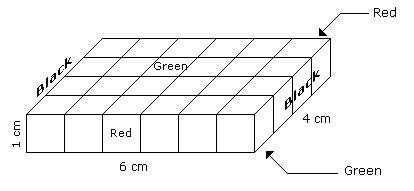# Verbal Reasoning - Cube and Cuboid - Discussion

Discussion Forum : Cube and Cuboid - Cube and Cuboid 1 (Q.No. 5)
Directions to Solve

The following questions are based on the information given below:

1. A cuboid shaped wooden block has 6 cm length, 4 cm breadth and 1 cm height.
2. Two faces measuring 4 cm x 1 cm are coloured in black.
3. Two faces measuring 6 cm x 1 cm are coloured in red.
4. Two faces measuring 6 cm x 4 cm are coloured in green.
5. The block is divided into 6 equal cubes of side 1 cm (from 6 cm side), 4 equal cubes of side 1 cm(from 4 cm side).

5.
How many cubes will remain if the cubes having black and green coloured are removed ?
4
8
12
16
Explanation:Number of small cubes which are Black and Green is 8 in all.

Hence, the number of remaining cubes are = 24 - 8 = 16

Discussion:
13 comments Page 2 of 2.

Chaitanya Reddy.M said:   1 decade ago
Here 6x4 small cubes are there so from top view from one side 2 small cubes and from another side 2cubes that is total 4cubes from top view and from bottom view their are 4cube total 8 cubes which are having only two colors black and green,those are removed from the total number of cube that is 24-8=16.

So ans is 16 cubes

All no of cubes which colored by green and black both = 8.

Because black colored in left and right both sides,

When we remove these two sides we will have only. 16 small cubes.

Those colored only with red & green.

Do you understand shilpi.